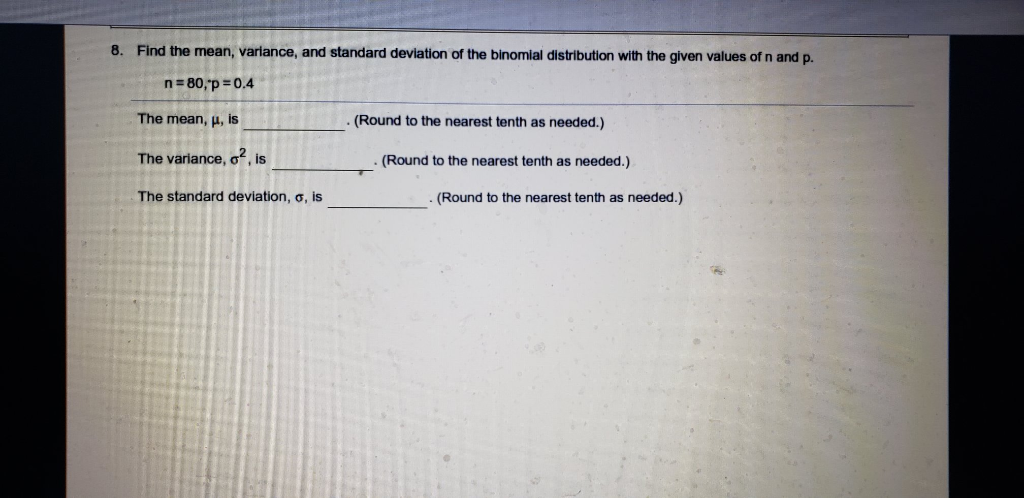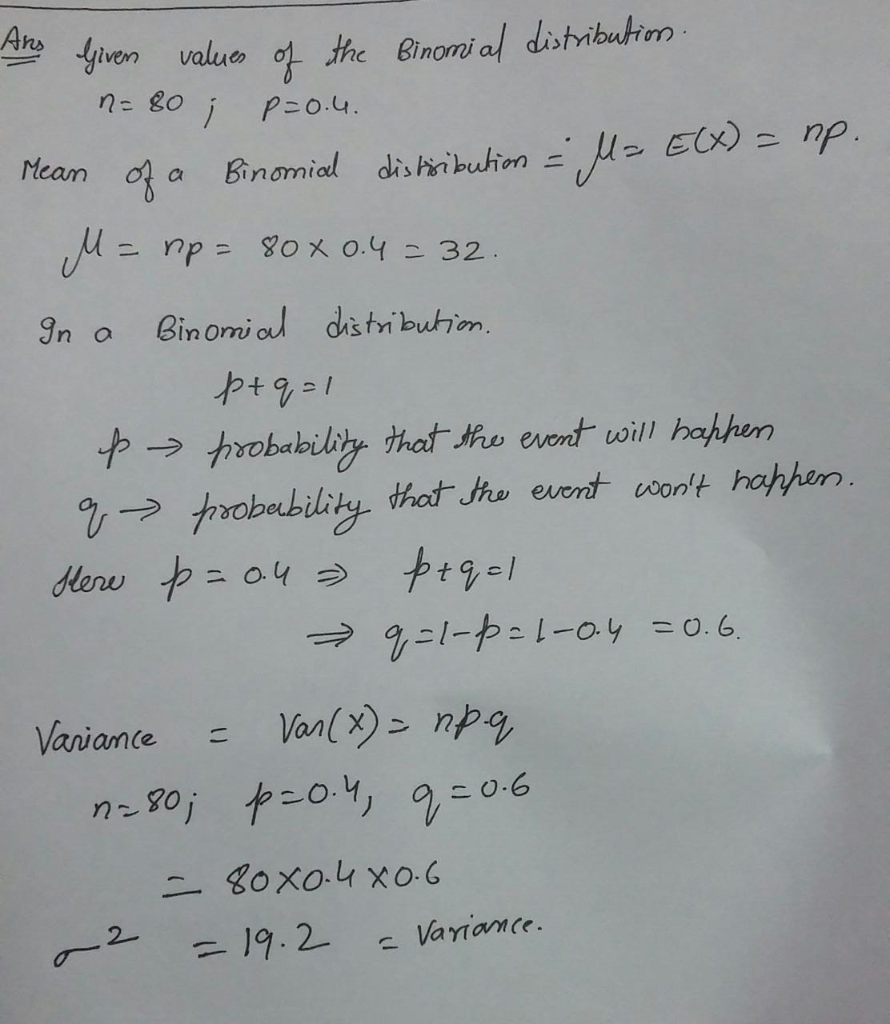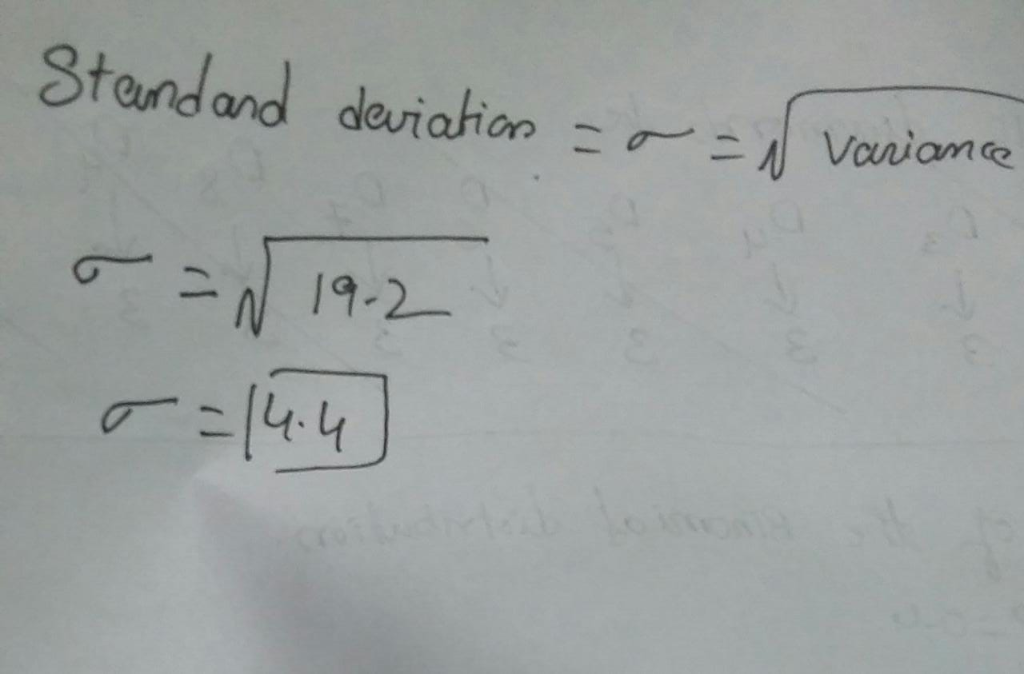# 8. Find the mean, variance, and standard deviation of the binomial distribution with the given values of n and p. n 80,...8. Find the mean, variance, and standard deviation of the binomial distribution with the given values of n and p. n 80, p 0.4 The mean, u, is (Round to the nearest tenth as needed.) The variance, o, is (Round to the nearest tenth as needed.) The standard deviation, G, is (Round to the nearest tenth as needed.)##### Add Answer of: 8. Find the mean, variance, and standard deviation of the binomial distribution with the given values of n and p. n 80,...
More Homework Help Questions Additional questions in this topic.